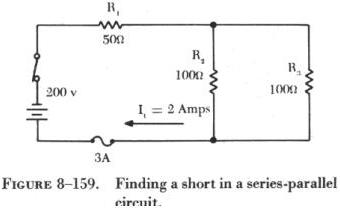BASICCIRCUIT2 BASIC CIRCUIT ANALYSIS AND TROUBLESHOOTING

Troubleshooting is the process of locating causes for malfunctions or trouble in a circuit. The following definitions serve as a guide in the troubleshooting discussion:

(1) Short circuit - a low resistance path. It can be across the power source or between the sides of a circuit. It usually creates high current flow which will burn out or cause damage to the circuit conductor or components.

(2) Open circuit - a circuit that is not complete or continuous.

(3) Continuity - the state of being continuous, or connected together; said of a circuit that is not broken or does not have an open.

(4) Discontinuity - the opposite of continuity, indicating that a circuit is broken or not continuous.

Figure 8-141 includes some of the most common sources of open circuits (commonly called "opens" or "an open"). A loose connection or no connection is a frequent cause of an open circuit. In A of figure 8-141, the end of a conductor has separated from the battery terminal. This type of malfunction opens a circuit and stops the flow of current. Another type of malfunction that will cause an open circuit is a burned out resistor, shown in B of figure 8-141. When a resistor overheats, its resistance value changes; and, if the current flow through it is great enough, it can burn and open the circuit. In C, D, and E of figure 8-141, three more likely causes of open circuits are shown.

 The opens shown can often be located by visual inspection; however, many circuit opens cannot be seen. In such cases, a meter must be used. The circuit shown in figure 8-142 is designed to cause current to flow through a lamp, but because of the open resistor, the lamp will not light. To locate this open, a voltmeter or an ohmmeter can be used.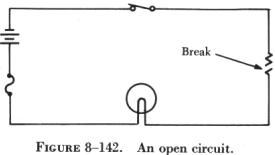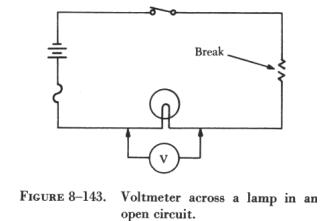If a voltmeter is connected across the lamp, as shown in figure 8-143, the voltmeter will read zero. Since no current can flow in the circuit because of the open resistor, there is no voltage drop across the lamp. This illustrates a troubleshooting rule that should be remembered: When a voltmeter is connected across a good (not defective) component in an open circuit, the voltmeter will read zero. Next, the voltmeter is connected across the open resistor, as shown in figure 8-144. The voltmeter has closed the circuit by shunting (paralleling) the burned out resistor, allowing current to flow. Current will flow from the negative terminal of the battery, through the switch, through the voltmeter and the lamp, back to the positive terminal of the battery. However, the resistance of the voltmeter is so high that only a very small current flows in the circuit. The current is too small to light the lamp, but the voltmeter will read the battery voltage. Another troubleshooting point worth remembering is: When a voltmeter is placed across an open component in a series circuit, it will read the battery, or applied voltage.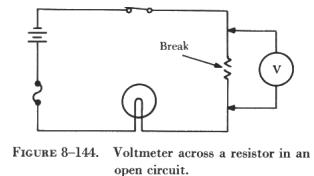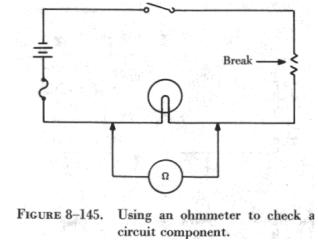This type of open circuit malfunction can also be traced by using an ohmmeter. When an ohmmeter is used, the circuit component to be tested must be isolated and the power source removed from the circuit. In this case, as shown in figure 8-145, these requirements can be met by opening the circuit switch. The ohmmeter is zeroed and placed across (in parallel with) the lamp. In this circuit, some value of resistance is read. This illustrates another important troubleshooting point: when an ohmmeter is properly connected across a circuit component and a resistance reading is obtained, the component has continuity and is not open. When the ohmmeter is connected across the open resistor, as shown in figure 8-146, it indicates infinite resistance, or a discontinuity. Thus, the circuit open has been located with both a voltmeter and an ohmmeter. An open in a series circuit will cause the current flow to stop. A short circuit, or "short," will cause the opposite effect. A short across a series circuit produces a greater than normal current flow. Some examples of shorts, as shown in figure 8-147, are two bare wires in a circuit that are touching each other, two terminals of a resistor connected together, etc. Thus, a short can be described as a connection of two conductors of a circuit through a very low resistance.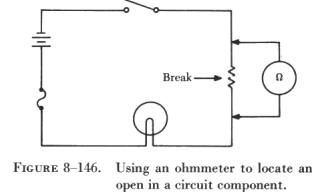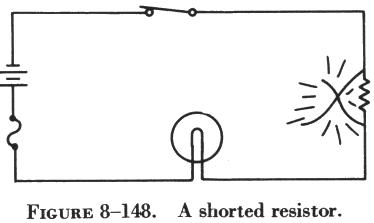In figure 8-148, a circuit is designed to light a lamp. A resistor is connected in the circuit to limit current flow. If the resistor is shorted, as shown in the illustration, the current flow will increase and the lamp will become brighter. If the applied voltage were high enough, the lamp would burn out, but in this case the fuse would protect the lamp by opening first. Usually a short circuit will produce an open circuit by either blowing (opening) the fuse or burning out a circuit component. But in some circuits, such as that illustrated in figure 8-149, there may be additional resistors which will not allow one shorted resistor to increase the current flow enough to blow the fuse or burn out a component. Thus, with one resistor shorted out, the circuit will still function since the power dissipated by the other resistors does not exceed the rating of the fuse. To locate the shorted resistor while the circuit is functioning, a voltmeter could be used. When it is connected across any of the unshorted resistors, a portion of the applied voltage will be indicated on the voltmeter scale. When it is connected across the shorted resistor, the voltmeter will read zero.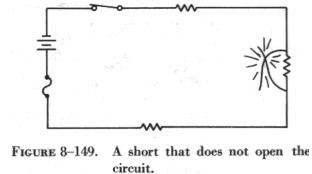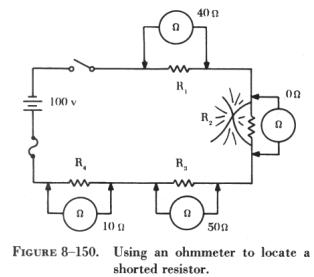The shorted resistor shown in figure 8-150 can be located with an ohmmeter. First the switch is opened to isolate the circuit components. In figure 8-150, this circuit is shown with an ohmmeter connected across each of the resistors. Only the ohmmeter connected across the shorted resistor shows a zero reading, indicating that this resistor is shorted. The procedures used in troubleshooting a parallel circuit are sometimes different from those used in a series circuit. Unlike a series circuit, a parallel circuit has more than one path in which current flows. A voltmeter cannot be used, since, when it is placed across an open resistor, it will read the voltage drop in a parallel branch. But an ammeter or the modified use of an ohmmeter can be employed to detect an open branch in a parallel circuit.

If the open resistor shown in figure 8-151 was not visually apparent, the circuit would appear to be functioning properly, since current would continue to flow in the other two branches of the circuit. To determine that the circuit is not operating properly, the total resistance, total current, and the branch currents of the circuit should be calculated as if there were no open in the circuit: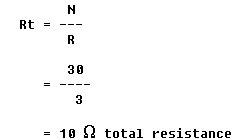Since the voltage applied to the branches is the same and the value of each branch resistance is known,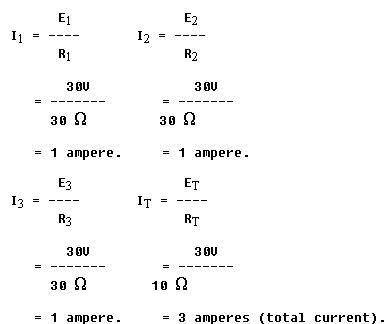An ammeter placed in the circuit to read total current would show 2 amperes instead of the calculated 3 amperes. Since 1 ampere of current should be flowing through each branch, it is obvious that one branch is open. If the ammeter is connected into the branches, one after another, the open branch will be located by a zero ammeter reading.

 A modified use of the ohmmeter can also locate this type of open. If the ohmmeter is connected across the open resistor, as shown in figure 8-152, an erroneous reading of continuity would be obtained. Even though the circuit switch is open, the open resistor is still in parallel with R1 and R2, and the ohmmeter would indicate the open resistor had a resistance of 15 ohms, the equivalent resistance of the parallel combination of R1 and R2. Thus, it is necessary to open the circuit as shown in figure 8-153 in order to check the resistance of R3. In this way the resistor is not shunted (paralleled) and the reading on the ohmmeter will indicate infinite resistance. On the other hand, if an open should occur in this circuit (figure 8-153) between the battery and point A, or between the battery and point B, current would not flow in the circuit.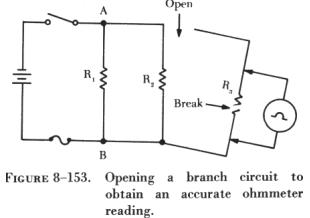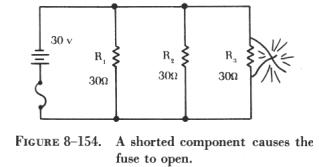As in a series circuit, a short in a parallel circuit will usually cause an open circuit by blowing the fuse. But, unlike a series circuit, one shorted component in a parallel circuit will stop current flow by causing the fuse to open. This can be seen by referring to the circuit in figure 8-154. If resistor R3 is shorted, a path of almost zero resistance will be offered the current, and all the circuit current will flow through the branch containing the shorted resistor. Since this is practically the same as connecting a wire between the terminals of the battery, the current will rise to an excessive value, and the fuse will open.
 Since the fuse opens almost as soon as a resistor shorts out, there is no time to perform a current or voltage check. Thus, troubleshooting a parallel dc circuit for a shorted component should be accomplished with an ohmmeter. But, as in the case of checking for an open resistor in a parallel circuit, a shorted resistor can be detected with an ohmmeter only if one end of the shorted resistor is disconnected. Troubleshooting a series-parallel resistive circuit involves locating malfunctions similar to those found in a series or a parallel circuit. In the circuit shown in figure 8-155, an open has occurred in the series portion of the circuit. When an open occurs anywhere in the series portion of a series-parallel circuit, current flow in the entire circuit will stop. In this case, the circuit will not function, and the lamp, L1, will not be lit.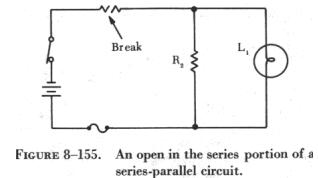If an open occurs in the parallel portion of a series-parallel circuit, as shown in figure 8-156, part of the circuit will continue to function. In this case, the lamp will continue to burn, but its brightness will diminish, since the total resistance of the circuit has increased and the total current has decreased.

If a break occurs in the branch containing the lamp, as shown in figure 8-157, the circuit will continue to function with increased resistance and decreased current, but the lamp will not burn.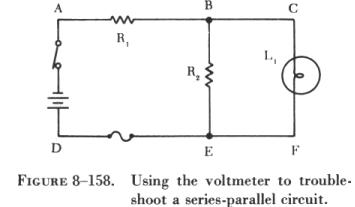To explain how the voltmeter and ohmmeter can be used to troubleshoot series-parallel circuits, the circuit shown in figure 8-158 has been labeled at various points. By connecting a voltmeter between points A and D, the battery and switch can be checked for opens. By connecting the voltmeter between points A and B, the voltage drop across R1 can be checked. This voltage drop is a portion of the applied voltage. Also, if R1 is open, the reading between B and D will be zero. The conductor between the positive terminal of the battery and point E, as well as the fuse, can be checked for continuity by connecting the voltmeter between points A and E. If the conductor or fuse is open, the voltmeter will read zero.

If the lamp is burning, it is obvious that no open exists in the branch containing the lamp, and the voltmeter could be used to detect an open in the branch containing R2 by removing lamp, L1, from the circuit.

Troubleshooting the series portion of a series-parallel circuit presents no difficulties, but in the parallel portion of the circuit misleading readings can be obtained.

An ohmmeter can be used to troubleshoot this same circuit. With the switch open, the series portion of the circuit can be checked by placing the ohmmeter leads between points A and B. If R1 or the conductor is open, the ohmmeter will read infinity; if not, the value of the resistor will be indicated on the ohmmeter. Between points D and E the fuse and conductor can be checked for continuity, but in the parallel portion of the circuit, care must be exercised, since misleading ohmmeter indications can be obtained. To check between points B and E, the branch must be disconnected at one of these points, and while one of these points and the switch are open, the branch containing the lamp can be cheeked with the ohmmeter.

 A short in the series part of a series-parallel circuit will cause a decrease in total resistance, which will cause total current to increase. In the circuit shown in figure 8-159, the total resistance is 100 ohms and the total current is 2 amperes. If R1 became shorted, total resistance would become 50 ohms, and the total current would double to 4 amperes. In the circuit shown, this would cause the 3 amp fuse to blow, but with a 5 amp fuse the circuit would continue to function. The result would be the same if R2 or R3 were to become shorted. The total resistance in either case would drop to 50 ohms. From this, it can be stated that when a short occurs in a series-parallel circuit, the total resistance will decrease and the total current will increase. A short will normally cause an open circuit by either blowing the fuse or burning out a circuit component. And, as in the case of an open, a short in a series-parallel circuit can be detected with either an ohmmeter or a voltmeter.